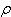From: Debra, a parent How much does 1300cc of liquid weigh, and how is the formula equated? Hi Debra. The answer is: it depends. Cubic centimeters (abbreviated "cc" and equivalent to millilitres, "ml") are a volume measurement. To convert that to weight (or more precisely, mass), you need to know the density and density is something specific to the type of liquid. The density of pure water is 1 gram per cc, so 1300 cc of H2 O would weigh 1.3 kg. Notice that olive oil always floats on water. That's because its density is lower than that of water - about 0.9 g / cc. Things that are less dense always float above those that are more dense. There's another scientific term you may have heard: "specific gravity". It's an odd name: it just means the ratio of the density of the substance to the density of water. So the specific gravity of olive oil is about 0.9. If you know the specific gravity, you know the density. The greek symbol(rho) represents density. So the formula is m = V, where m is the mass and V is the volume. Hope this helps, Stephen La Rocque.